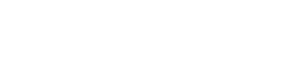Skriv utLukk vindu

MAT-3200 Mathematical Methods - 10 stp

The course is administrated by

Faculty of Science and Technology

Type of course

The course is mandatory in the study program Mathematics and Finance - master, and it's included in the study programs Mathematics - master and Applied Physics and Mathematics - master (5-years). It may also be taken independent of study program upon approval of the Department of mathematics and statistics.

Course contents

This is a course on the mathematical modeling of linear continuous systems. These are systems containing an infinite number of degrees of freedom. Examples taken from the modeling of traffic flow, heat conduction, waves and option pricing in economy (Blach Scholes formula). The course will cover both analytic and numerical methods and will give an introduction to the numerical solution of partial differential equations with emphasis on the basic model equations derived in the course. The course MAT-2200, Differential equations, is a prerequisite and some familiarity with numerical methods is highly recommended. Some knowledge of physics would also be helpful.

Applicants from Nordic countries: 1 June for the autumn semester and 1 December for the spring semester. Applicants from outside the Nordic countries: 1 October for the spring semester and 15 April for the autumn semester.

Bachelor of science degree in mathematics or equal, including our course MAT-2200 Differential Equations or other equivalent course in differential equations.
Application code is 9371.

Objective of the course

After the course the students are supposed to know

• Simple models leading to central partial differential equations
• First order partial differential equations
• Classification and solution methods for second order partial differential equations
• Separation of variables and generalized Fourier series
• integral transforms
• Green’s functions and og distributions
• Finite difference methods for partial differential equations

After the course the students are expected to be able to apply both traditional analytic solutions methods and numerical methods to solve partial differential equations.

Language of instruction

The language of instruction and the syllabus is English. Examination questions will be given in English, but may be answered either in English or a Scandinavian language.

Lectures: 40 h
Coursework: 30 h

Assessment

One oral exam counting 100%.

Assessment scale: Letter grades A-F.

Coursework requirements
A passing grade is required on the mandatory homework sets for permission to take the exam.